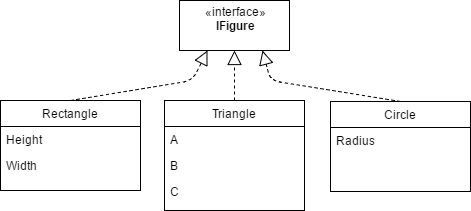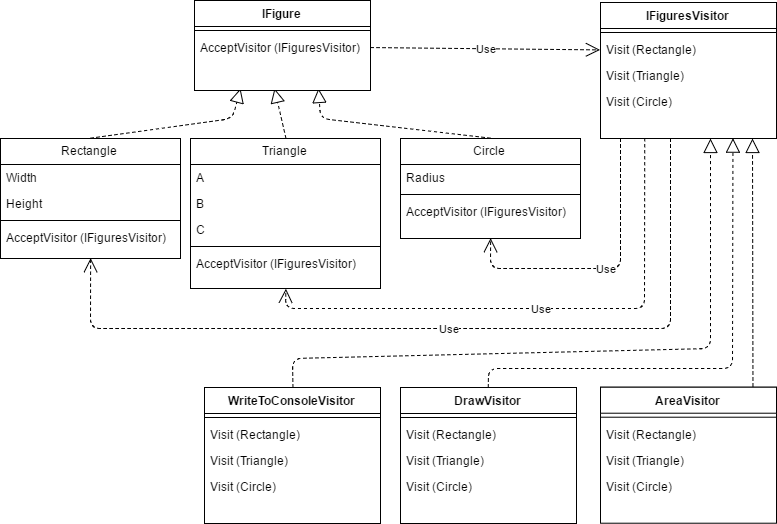14:30

# Drilling Down the Visitor Pattern

Recently, I have often had to use the well-known Visitor pattern. I used to ignore this pattern and thought that it simply complicates the code. In this article, I will share my thoughts about this pattern. We will talk about pros and cons, as well as what tasks it helps to solve and how to simplify its use. The code will be in C#.

## What is it?

Assume we have a library with a hierarchy of geometric shapes.Now, we need to learn how to calculate the areas of these shapes. No problem! We can add the method to IFigure and implement it. Everything is fine, except that now our library depends on the library of algorithms.

Later, we may need to display the description of each shape in the console and draw the shapes. By adding appropriate methods, we inflate our library, incidentally violating SRP and OCPs.

What can we do? Of course, we need to create classes in individual libraries that solve required tasks. How will these classes know which particular shape was passed? The answer is a type casting.

```public void Draw(IFigure figure)
{
if (figure is Rectangle)
{
///////
return;
}
if (figure is Triangle)
{
///////
return;
}
if (figure is Triangle)
{
///////
return;
}
}```

Have you seen the error? I noticed one only at runtime. Downcasting is a badly considered design, a way to violate LSP, etc. There are programming languages, that provide (multi-methods), but C# is not among them.

Here the Visitor pattern comes into action. The point is that there is a class called Visitor that contains methods for working with each of the specific implementations of our abstraction. Each particular implementation contains a method that passes itself to the appropriate Visitor method.It is a little bit confusing, isn’t it? The Visitor pattern is quite complex to understand on the first try, which is its main drawback. Using this template increases the complexity of the system.

As you can see, all the logic is in visitors, rather than in our geometric shapes. There is no typecasting at runtime. The method for each shape is defined at compile time. Thus, we have resolved the problems we had faced before. However, there are some drawbacks, which I am going to discuss later.

## Use cases

What value type should the Visit and Acceptvisitor methods return? In the classic version, they are void.

What do we need to do when calculating areas of shapes? Well, we can declare a property inside Visitor and assign a value to it. Then, after we call Visit, we can read that property.

However, it would be more convenient if the Acceptvisitor method could return the result immediately.

In this case, the return type is double, but it depends.

Let’s make Visitor and Acceptvisitor generic methods.

```public interface IFigure
{
T AcceptVisitor<T>(IFiguresVisitor<T> visitor);
}

public interface IFiguresVisitor<out T>
{
T Visit(Rectangle rectangle);
T Visit(Triangle triangle);
T Visit(Circle circle);
}```

This interface can be used in all cases. For asynchronous operations, the return type will be Task. If nothing needs to be returned, the return type can be blank, available in the functional languages as Unit. In C #, it is also defined in some libraries (e.g. Reactive Extensions).

There are situations when, depending on the object type, we need to perform a trivial action, just in one place of the program. For example, in practice, we are unlikely to display the name of a shape anywhere except in the test case. Alternatively, in any unit test, it would be required to determine that the shape is a circle or a rectangle.

Alternatively, in a unit test, it is necessary to determine whether the figure is a circle or a rectangle.

So, do we really need to create a new entity (a specialized visitor) for each primitive case? You can do the following:

```public class FiguresVisitor<T> : IFiguresVisitor<T>
{

public FiguresVisitor(Func<Rectangle, T> ifRectangle, Func<Triangle, T> ifTrian-gle, Func<Circle, T> ifCircle)
{
_ifRectangle = ifRectangle;
_ifTriangle = ifTriangle;
_ifCircle = ifCircle;
}

public T Visit(Rectangle rectangle) => _ifRectangle(rectangle);

public T Visit(Triangle triangle) => _ifTriangle(triangle);

public T Visit(Circle circle) => _ifCircle(circle);
}```
```public double CalcArea(IFigure figure)
{
var visitor = new FiguresVisitor<double>(
r => r.Height * r.Width,
t =>
{
var p = (t.A + t.B + t.C) / 2;
return Math.Sqrt(p * (p - t.A) * (p - t.B) * (p - t.C));
},

return figure.AcceptVisitor(visitor);
}```

As you can see, we got something that resembles pattern matching, not the one that was added in C # 7, which is essentially just this Downcasting, but typed and controlled by the compiler.

What if we have a dozen of shapes? We only need to perform a particular operation for one or two while for the others it is necessary to perform a default action. Do we need to copy dozens of identical expressions to the constructor? It seems that it is not the best approach. What about the following syntax?

```string description = figure
.IfRectangle(r => \$"Rectangle with area={r.Height * r.Width}")
.Else(() => "Not rectangle");```
```bool isCircle = figure
.IfCircle(_=>true)
.Else(() => false);```

In the last example, we get the true equivalent of the “is” operator! You may find the implementation of this factory for our set of shapes on GitHub.

The following question arises. Do we need to write this boilerplate for every single case? Yes, we do. Alternatively, you can create a code generator with T4 and Roslyn.

There are many drawbacks and limitations in Visitor. Let’s take a look at the AcceptVisitor method from iFigure. It has nothing to do with geometry. So again, I got SRP violation.

Look at the diagram again:We can see a closed system where everyone knows everything. Each type of the hierarchy knows about Visitor. Visitor knows about all types. Therefore, every type transitively knows about all the others! Adding a new type (shapes in our example) actually affects everyone, which is a direct violation of the previously mentioned Open Close Principle.

The ability to modify the code is a significant benefit. If we add a new shape, the compiler will make us add the appropriate method to the Visitor interface and its implementation. We won’t forget anything.

However, what if we are just users of the library and not the authors, and we can’t change the hierarchy?

We cannot expand someone else’s structure with Visitor. All the pattern definitions mention that it is applied when there is a well-established hierarchy. Therefore, if we design an extensible geometric shape library, we cannot use Visitor.

## Summary

The “Visitor” pattern is very useful when we have the ability to make changes to its code. It allows us to avoid Downcasting. Its “inextensibility” allows the compiler to ensure that you add all handlers for all new types.

If you are going to write a library that can be extended by adding new types, the Visitor pattern will not help you. In this case, you can use Downcasting wrapped in the C# 7 Pattern matching or create something more interesting.